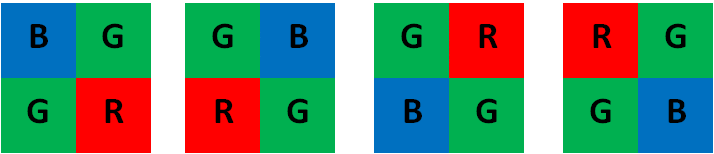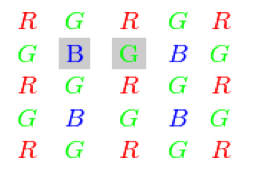OpenCV  3.4.20-dev Open Source Computer Vision
Color conversions
Todo:
document other conversion modes

## RGB $$\leftrightarrow$$ GRAY

Transformations within RGB space like adding/removing the alpha channel, reversing the channel order, conversion to/from 16-bit RGB color (R5:G6:B5 or R5:G5:B5), as well as conversion to/from grayscale using:

$\text{RGB[A] to Gray:} \quad Y \leftarrow 0.299 \cdot R + 0.587 \cdot G + 0.114 \cdot B$

and

$\text{Gray to RGB[A]:} \quad R \leftarrow Y, G \leftarrow Y, B \leftarrow Y, A \leftarrow \max (ChannelRange)$

The conversion from a RGB image to gray is done with:

cvtColor(src, bwsrc, cv::COLOR_RGB2GRAY);

More advanced channel reordering can also be done with cv::mixChannels.

cv::COLOR_BGR2GRAY, cv::COLOR_RGB2GRAY, cv::COLOR_GRAY2BGR, cv::COLOR_GRAY2RGB

## RGB $$\leftrightarrow$$ CIE XYZ.Rec 709 with D65 white point

$\begin{bmatrix} X \\ Y \\ Z \end{bmatrix} \leftarrow \begin{bmatrix} 0.412453 & 0.357580 & 0.180423 \\ 0.212671 & 0.715160 & 0.072169 \\ 0.019334 & 0.119193 & 0.950227 \end{bmatrix} \cdot \begin{bmatrix} R \\ G \\ B \end{bmatrix}$

$\begin{bmatrix} R \\ G \\ B \end{bmatrix} \leftarrow \begin{bmatrix} 3.240479 & -1.53715 & -0.498535 \\ -0.969256 & 1.875991 & 0.041556 \\ 0.055648 & -0.204043 & 1.057311 \end{bmatrix} \cdot \begin{bmatrix} X \\ Y \\ Z \end{bmatrix}$

$$X$$, $$Y$$ and $$Z$$ cover the whole value range (in case of floating-point images, $$Z$$ may exceed 1).

cv::COLOR_BGR2XYZ, cv::COLOR_RGB2XYZ, cv::COLOR_XYZ2BGR, cv::COLOR_XYZ2RGB

## RGB $$\leftrightarrow$$ YCrCb JPEG (or YCC)

$Y \leftarrow 0.299 \cdot R + 0.587 \cdot G + 0.114 \cdot B$

$Cr \leftarrow (R-Y) \cdot 0.713 + delta$

$Cb \leftarrow (B-Y) \cdot 0.564 + delta$

$R \leftarrow Y + 1.403 \cdot (Cr - delta)$

$G \leftarrow Y - 0.714 \cdot (Cr - delta) - 0.344 \cdot (Cb - delta)$

$B \leftarrow Y + 1.773 \cdot (Cb - delta)$

where

$delta = \left \{ \begin{array}{l l} 128 & \mbox{for 8-bit images} \\ 32768 & \mbox{for 16-bit images} \\ 0.5 & \mbox{for floating-point images} \end{array} \right .$

Y, Cr, and Cb cover the whole value range.

cv::COLOR_BGR2YCrCb, cv::COLOR_RGB2YCrCb, cv::COLOR_YCrCb2BGR, cv::COLOR_YCrCb2RGB

## RGB $$\leftrightarrow$$ HSV

In case of 8-bit and 16-bit images, R, G, and B are converted to the floating-point format and scaled to fit the 0 to 1 range.

$V \leftarrow max(R,G,B)$

$S \leftarrow \fork{\frac{V-min(R,G,B)}{V}}{if $$V \neq 0$$}{0}{otherwise}$

$H \leftarrow \forkfour{{60(G - B)}/{(V-min(R,G,B))}}{if $$V=R$$} {{120+60(B - R)}/{(V-min(R,G,B))}}{if $$V=G$$} {{240+60(R - G)}/{(V-min(R,G,B))}}{if $$V=B$$} {0}{if $$R=G=B$$}$

If $$H<0$$ then $$H \leftarrow H+360$$ . On output $$0 \leq V \leq 1$$, $$0 \leq S \leq 1$$, $$0 \leq H \leq 360$$ .

The values are then converted to the destination data type:

• 8-bit images: $$V \leftarrow 255 V, S \leftarrow 255 S, H \leftarrow H/2 \text{(to fit to 0 to 255)}$$
• 16-bit images: (currently not supported) $$V <- 65535 V, S <- 65535 S, H <- H$$
• 32-bit images: H, S, and V are left as is
cv::COLOR_BGR2HSV, cv::COLOR_RGB2HSV, cv::COLOR_HSV2BGR, cv::COLOR_HSV2RGB

## RGB $$\leftrightarrow$$ HLS

In case of 8-bit and 16-bit images, R, G, and B are converted to the floating-point format and scaled to fit the 0 to 1 range.

$V_{max} \leftarrow {max}(R,G,B)$

$V_{min} \leftarrow {min}(R,G,B)$

$L \leftarrow \frac{V_{max} + V_{min}}{2}$

$S \leftarrow \fork { \frac{V_{max} - V_{min}}{V_{max} + V_{min}} }{if $$L < 0.5$$ } { \frac{V_{max} - V_{min}}{2 - (V_{max} + V_{min})} }{if $$L \ge 0.5$$ }$

$H \leftarrow \forkfour {{60(G - B)}/{(V_{max}-V_{min})}}{if $$V_{max}=R$$ } {{120+60(B - R)}/{(V_{max}-V_{min})}}{if $$V_{max}=G$$ } {{240+60(R - G)}/{(V_{max}-V_{min})}}{if $$V_{max}=B$$ } {0}{if $$R=G=B$$ }$

If $$H<0$$ then $$H \leftarrow H+360$$ . On output $$0 \leq L \leq 1$$, $$0 \leq S \leq 1$$, $$0 \leq H \leq 360$$ .

The values are then converted to the destination data type:

• 8-bit images: $$V \leftarrow 255 \cdot V, S \leftarrow 255 \cdot S, H \leftarrow H/2 \; \text{(to fit to 0 to 255)}$$
• 16-bit images: (currently not supported) $$V <- 65535 \cdot V, S <- 65535 \cdot S, H <- H$$
• 32-bit images: H, S, V are left as is
cv::COLOR_BGR2HLS, cv::COLOR_RGB2HLS, cv::COLOR_HLS2BGR, cv::COLOR_HLS2RGB

## RGB $$\leftrightarrow$$ CIE L*a*b*

In case of 8-bit and 16-bit images, R, G, and B are converted to the floating-point format and scaled to fit the 0 to 1 range.

$\vecthree{X}{Y}{Z} \leftarrow \vecthreethree{0.412453}{0.357580}{0.180423}{0.212671}{0.715160}{0.072169}{0.019334}{0.119193}{0.950227} \cdot \vecthree{R}{G}{B}$

$X \leftarrow X/X_n, \text{where} X_n = 0.950456$

$Z \leftarrow Z/Z_n, \text{where} Z_n = 1.088754$

$L \leftarrow \fork{116*Y^{1/3}-16}{for $$Y>0.008856$$}{903.3*Y}{for $$Y \le 0.008856$$}$

$a \leftarrow 500 (f(X)-f(Y)) + delta$

$b \leftarrow 200 (f(Y)-f(Z)) + delta$

where

$f(t)= \fork{t^{1/3}}{for $$t>0.008856$$}{7.787 t+16/116}{for $$t\leq 0.008856$$}$

and

$delta = \fork{128}{for 8-bit images}{0}{for floating-point images}$

This outputs $$0 \leq L \leq 100$$, $$-127 \leq a \leq 127$$, $$-127 \leq b \leq 127$$ . The values are then converted to the destination data type:

• 8-bit images: $$L \leftarrow L*255/100, \; a \leftarrow a + 128, \; b \leftarrow b + 128$$
• 16-bit images: (currently not supported)
• 32-bit images: L, a, and b are left as is
cv::COLOR_BGR2Lab, cv::COLOR_RGB2Lab, cv::COLOR_Lab2BGR, cv::COLOR_Lab2RGB

## RGB $$\leftrightarrow$$ CIE L*u*v*

In case of 8-bit and 16-bit images, R, G, and B are converted to the floating-point format and scaled to fit 0 to 1 range.

$\vecthree{X}{Y}{Z} \leftarrow \vecthreethree{0.412453}{0.357580}{0.180423}{0.212671}{0.715160}{0.072169}{0.019334}{0.119193}{0.950227} \cdot \vecthree{R}{G}{B}$

$L \leftarrow \fork{116*Y^{1/3} - 16}{for $$Y>0.008856$$}{903.3 Y}{for $$Y\leq 0.008856$$}$

$u' \leftarrow 4*X/(X + 15*Y + 3 Z)$

$v' \leftarrow 9*Y/(X + 15*Y + 3 Z)$

$u \leftarrow 13*L*(u' - u_n) \quad \text{where} \quad u_n=0.19793943$

$v \leftarrow 13*L*(v' - v_n) \quad \text{where} \quad v_n=0.46831096$

This outputs $$0 \leq L \leq 100$$, $$-134 \leq u \leq 220$$, $$-140 \leq v \leq 122$$ .

The values are then converted to the destination data type:

• 8-bit images: $$L \leftarrow 255/100 L, \; u \leftarrow 255/354 (u + 134), \; v \leftarrow 255/262 (v + 140)$$
• 16-bit images: (currently not supported)
• 32-bit images: L, u, and v are left as is

Note that when converting integer Luv images to RGB the intermediate X, Y and Z values are truncated to $$[0, 2]$$ range to fit white point limitations. It may lead to incorrect representation of colors with odd XYZ values.

The above formulae for converting RGB to/from various color spaces have been taken from multiple sources on the web, primarily from the Charles Poynton site http://www.poynton.com/ColorFAQ.html

cv::COLOR_BGR2Luv, cv::COLOR_RGB2Luv, cv::COLOR_Luv2BGR, cv::COLOR_Luv2RGB

## Bayer $$\rightarrow$$ RGB

The Bayer pattern is widely used in CCD and CMOS cameras. It enables you to get color pictures from a single plane where R, G, and B pixels (sensors of a particular component) are interleaved as follows:Bayer patterns (BGGR, GBRG, GRGB, RGGB)

The output RGB components of a pixel are interpolated from 1, 2, or 4 neighbors of the pixel having the same color.

Note
See the following for information about correspondences between OpenCV Bayer pattern naming and classical Bayer pattern naming.Bayer pattern

There are several modifications of the above pattern that can be achieved by shifting the pattern one pixel left and/or one pixel up. The two letters $$C_1$$ and $$C_2$$ in the conversion constants CV_Bayer $$C_1 C_2$$ 2BGR and CV_Bayer $$C_1 C_2$$ 2RGB indicate the particular pattern type. These are components from the second row, second and third columns, respectively. For example, the above pattern has a very popular "BG" type.